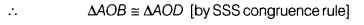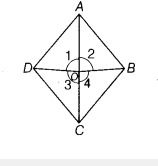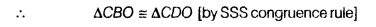# In a quadrilateral ABCD, there is a point O inside it such that OB = OD

In a quadrilateral ABCD, there is a point O inside it such that OB = OD. Also, AB = AD and BC = DC. Prove that O lies on AC

In ∆AOB and ∆AOD,
OB=OD
OA=OA∠1 = ∠2Similarly, in ∆CBO and ∆CDO,
BC = DC [given]
OB = OD [given]
and OC = OC [common side]∠3 = ∠4
On adding Eqs. (i) and (ii), we get ∠1+∠3 = ∠2 +∠4

We know that∠1 +∠3+∠2 +∠4 = 360

[∴ sum of all angles around a point is 360°]
∠1 + ∠3+ ∠1 + ∠3 = 360° [from Eqs. (I) and (ii)]
2(∠1+∠3) = 360°
∠1+∠3 = 180°
∠AOC = 180°

Hence, O lies on AC.